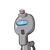# 2. P = Rs. 3000, R = 9%, T = 2½y, then I = _____________ ( )A) Rs. 575 B) Rs. 685 C) Rs. 655 D) Rs. 675​

2. P = Rs. 3000, R = 9%, T = 2½y, then I = _____________ ( )

A) Rs. 575 B) Rs. 685 C) Rs. 655 D) Rs. 675​

### 1 thought on “2. P = Rs. 3000, R = 9%, T = 2½y, then I = _____________ ( )<br /><br />A) Rs. 575 B) Rs. 685 C) Rs. 655 D) Rs. 675​”

1.